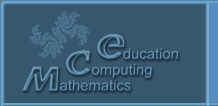Русский
 ! Вопросы и ответы

## Presentations

### About teaching discipline "Numerical methods" with use of the system Mathematica

Tokareva N.A., Ivantsova O.V., Ryseva O.A.

Dubna State University, 141982, Dubna Moscow region, Universitetskaya 19

The role of the specialized mathematical software systems in the process of teaching mathematical disciplines to students of IT areas is currently increasing. The aspects of teaching the discipline "Numerical methods" for students in the fields of "Informatics and computer engineering", "Information systems and technologies", "Applied Informatics" with the use of the Mathematica system at the Dubna state University are discussed. The authors present the structure and content of the practicum on the discipline. Each course unit contains theoretical references on topics and demonstration examples illustrating lecture materials.

The practicum includes the following units: "Introduction. Basic concepts"; "Numerical methods of linear algebra"; "Solution of nonlinear equations and systems"; "Approximation of functions"; "Numerical differentiation and integration" and "Solution of differential equations". In the "Introduction" section, examples of computational error accumulation and ill-conditioned problems are demonstrated. By means of Mathematica matrix operations in the next section the step by step algorithms of direct methods for solving the system of linear algebraic equations using LU and QR decompositions of the matrix of the system are described. It is also discussed here Danilevsky method and the iterative method for solving the algebraic eigenvalue problem. The stages of localization of the roots of the nonlinear equation, identification of the conditions of convergence of the iterative method and the organization of the converging iteration process are demonstrated by the examples of solving nonlinear equations. The function approximations section shows various methods of approximation and uses symbolic computation capabilities to derive equations that arise in the construction of polynomial interpolation splines. Euler, Runge-Kutta and Adams methods are used to solve differential equations. The ideas of obtaining Adams method calculation formulas of different order are realized by means of symbolic calculations. Each section includes tasks for self-solution.

Using the possibilities of symbolic calculations in Mathematica to obtain calculation formulas of methods allows not to overload lectures with routine calculations, but to pay more attention to the comparative analysis of methods and to justification of the choice of a particular method when solving a subject problem.

© 2004 Designed by Lyceum of Informational Technologies №1533